# KVPY-SA 2017 Mathematics Paper with Solutions

Students can find the KVPY-SA 2017 Maths paper solutions on this page. These step by step solutions are done by subject experts at BYJU’S. Solving these papers will help students to find out their weaker areas and give them an idea about which topics to concentrate more on. The PDF format of the solutions is also available for free. Revising these solutions will help students to improve their speed and accuracy.

### KVPY SA 2017 - Maths

Question 1: A quadrilateral has distinct integer side lengths. If the second-largest side has length 10, then the maximum possible length of the largest side is

1. a. 25
2. b. 26
3. c. 27
4. d. 28

Solution:

Let a, b, c, d are the four sides of a quadrilateral

Where a > b > c > d are distinct positive integers and b = 10 (given)

Now, we know that in a quadrilateral

Sum of any three sides > 4th side

⇒ a < b + c + d

Since b = 10 and b > c > d, so the largest possible values of c and d are 9 and 8 respectively.

⇒ a < 10 + 9 + 8

⇒ a < 27

Therefore, the largest possible value of a is 26.

Question 2: The largest power of 2 that divides 200!/100!

1. a. 98
2. b. 99
3. c. 100
4. d. 101

Solution:

The exponent of 2 in 200!

= (200/2) + (200/22) + ……… + (200/27)

= 100 + 50 + 12 + 6 + 3 + 1

= 197.

The exponent of 2 in 100!

= (100/2)+(100/22)+…(100/26)

= 50+12+6+3+1

= 97

Therefore, the exponent of 2 in 200!/100! = 197 – 97 = 100.

Question 3: Let a1, a2, a3, a4 be real numbers such that a1+ a2 +a3 + a4 = 0 and a12+ a22 +a32 + a42 = 1. Then the smallest possible value of the expression (a1 – a2)2 + (a2 – a3)2 + (a3 – a4)2 + (a4 – a1)2 lies in the interval

1. a. (0, 1.5)
2. b. (1.5, 2.5)
3. c. (2.5, 3)
4. d. (3, 3.5)

Solution:

Given, a12+ a22 +a32 + a42 = 1.

Therefore (a1 – a2)2 + (a2 – a3)2 + (a3 – a4)2 + (a4 – a1)2 = 0

When a1 = a2 = a3 = a4 = +1/2 or -1/2.

Question 4: Let S be the set of all ordered pairs (x, y) of positive integers satisfying the condition x2 – y2 = 12345678. Then

1. a. S is an infinite set
2. b. S is the empty set
3. c. S has exactly one element
4. d. S is a finite set and has at least two elements

Solution:

x2 – y2 = 12345678

OR

(x – y) (x + y) = 12345678 x, y belongs to z+

Case I: x, y belongs to odd

Difference of squares of two odd integers is multiple of 8 (or divisible by 8)

⇒ x2 – y2 = 8k, k belongs to I

But R.H.S is not divisible by ‘8’

Therefore, equality can’t hold.

Case II:x, y belongs to even

⇒ x – y belongs to even, x + y belongs to even

L.H.S = (x – y) (x + y)

= 2n×2m (n, m belongs to I)

= 4 mn (multiple of 4)

But R.H.S is not divisible by ‘4’

Again not possible.

Case III:x belongs to odd, y belongs to even

⇒ x – y belongs to odd, x + y belongs to odd

⇒ L.H.S = (x – y) (x + y)

= odd × odd

= odd

But R.H.S is even.

So not possible.

Therefore, S is an empty Set.

Question 5: Let A1A2A3....A9 be a nine-sided regular polygon with side length 2 units. The difference between the lengths of the diagonals A1A5 and A2A4 equals

1. a. 2+ √12
2. b. √12-2
3. c. 6
4. d. 2

Solution: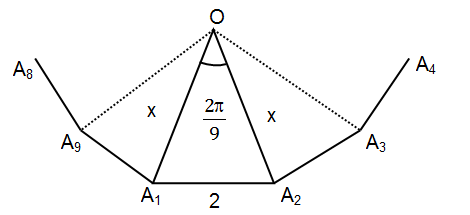In ΔA1OA2

∠ A1OA2 = 2 π/9 = 400

⇒ cos 40° = (x2+x2-22)/2.x.x {using cosine rule}

⇒ 2x2 (cos 400–1) = –4

⇒ x2 = 2/(1-cos 400) = 2/2sin2200

⇒ x = 1/sin 200 ……(1)

In Δ A1OA5

Now, ∠ A1OA5 = 8π/9

⇒ cos 8π/9 = (x2+x2-(A1A5)2)/2x2 {using cosine rule}

⇒ A1A52 = 2x2 (1 – cos 1600)

⇒ A1A5 = 2 x sin800 ……. (ii)

Similarly, In Δ A2OA4

A2A4 = 2 x sin400

Therefore A1A5 – A2A4 = 2 x (sin 800 – sin400)

= 2(1/sin 200)2.sin 200cos 600 (sin C – sin D = 2 cos(C+D)/2 sin (C-D)/2)

= 2

Question 6: Let a1, a2,.... an be n nonzero real numbers, of which p are positive and remaining are negative. The number of ordered pairs (j, k), j < k, for which aj ak is positive, is 55. Similarly, the number of ordered pairs (j, k), j < k, for which aj ak is negative is 50. Then the value of p2 + (n – p)2 is

1. a. 629
2. b. 325
3. c. 125
4. d. 221

Solution:

Given, a1, a2, .... an be n nonzero real numbers, among which p are positive and n – p are negative.

The number of ordered pair (j,k), j < k such that the product aj . ak is positive is 55.

Which is possible when either both aj, ak are positive or both are negative.

pC2 + n–p C2 = 55 (No. of ways of selecting two positive and two negative numbers)

p(p-1)/2 +(n-p)(n-p-1)/2 = 55

p2 + (n – p)2 – n = 55 ….(i)

The number of ordered pair (j,k), j < k such that the product aj . ak is negative is 50.

Which is possible when one of aj, ak is positive and other negative.

Required number of ways = Total number of ways of selecting two numbers - No. of

ways of selecting two positive and two negative numbers

nC2 – 55 = 50

n(n-1)/2 = 105

n2 – n – 210 = 0

n = 15, –14 (n can’t be negative therefore – 14 is rejected)

Now, put n = 15 in equation (i)

So p2 + (n – p)2 = 125

Question 7: If a, b, c, d are four distinct numbers chosen from the set {1,2,3 ....,9}, then the minimum value of is

1. a. 3/8
2. b. 1/3
3. c. 13/36
4. d. 25/72

Solution:

a, b, c, d ∈ {1, 2, 3, ………9}

Now, (a/b)+(c/d) is minimum if (a/b) and (c/d) individually become minimum.

a/b -> min, when a -> min and b -> max.

c/d -> min, when c -> min and d -> max.

Also a, b, c, d are distinct

So minimum (a/b)+(c/d) = (1/9)+(2/8) Or (1/8)+(2/9)

= 26/72 or 25/72

Question 8: If 72x. 48y = 6xy, where x and y are non zero rational numbers, then x + y equals

1. a. 3
2. b. 10/3
3. c. –3
4. d. –10/3

Solution:

72x. 48y = 6 x. y

(23.32)x.(24.31)y = (2.3)x.y

23x+ 4y. 32x + y = 2x. y . 3xy

Equating the powers of 2 and 3, we get

3x + 4y = xy …………(i)

2x + y = xy ……………(ii)

Subtract (ii) from (i)

x = – 3y { put in (i) }

3(–3y)+ 4y = (–3y).y

– 5y = – 3y2

y = 5/3, x = – 5

Hence x + y = (5/3)-5

= -10/3

Question 9: Let AB be a line segment of length 2. Construct a semicircle S with AB as diameter. Let C be the midpoint of the arc AB. Construct another semicircle T external to the triangle ABC with chord AC as diameter. The area of the region inside the semicircle T but outside S is

1. a. π/2
2. b. 1/2
3. c. π/√2
4. d. 1/√2

Solution: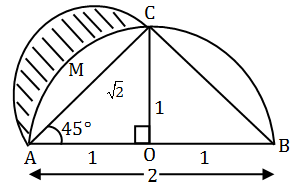C being midpoint of arc ACB ⇒ AX = BX ⇒ ∠CAB = ∠CBA

Also ∠ ACB = 90 (Angle in semicircle)

OC AB (Construction)

⇒ ∠OAC = ∠OCA = 45

OA =OX = 1

In ΔOAC

AC2 = OA2 + OC2

⇒ AC = √2

Now, Required Area = Area of semicircle on AC as diameter – Area of segment A.M.C.A

= (1/2) ×π (√2/2)2-((π /4)-(1/2) )

= ((π /4)-(π /4)+(1/2) )

= 1/2

Question 10: Let r(x) be the remainder when the polynomial x135 + x125 – x115 + x5 + 1 is divided by x3– x. Then.

1. a. r(x) is the zero polynomial
2. b. r(x) is a nonzero constant
3. c. degree of r(x) is one
4. d. degree of r(x) is two

Solution:

x135+ x125 – x115 + x5 + 1 = q(x) (x3 – x) + Ax2 +Bx + C

Where q(x) is quotient and r(x) = Ax2 +Bx + C is remainder

Now, putting x = 0, 1, –1

We get

⇒ 1 = C

⇒ 3 = A + B + C

⇒ A+B = 2 ….(i)

⇒ –1 = A – B + C

⇒ -1 = A-B+C

⇒ A - B = -2

⇒ 2A = 0

⇒ A = 0 and B = 2

So r(x) = 2x + 1 a polynomial of degree 1.

Question 11: It is given that the number 43361 can be written as a product of two distinct prime numbers p1 , p2. Further, assume that there are 42900 numbers which are less than 43361 and are co-prime to it. Then, p1+p2 is

1. a. 462
2. b. 464
3. c. 400
4. d. 402

Solution:

The prime factors given number are,

43361 = 131 × 331

p1 + p2 = 131 + 331

= 462

Question 12: Let ABC be a triangle with ∠ C = 900. Draw CD perpendicular to AB. Choose points M and N on sides AC and BC respectively such that DM is parallel to BC and DN is parallel to AC. If DM = 5, DN = 4, then AC and BC are respectively equal to

1. a. 41/4, 41/5
2. b. 39/4, 39/5
3. c. 38/4, 38/5
4. d. 37/4, 37/5

Solution: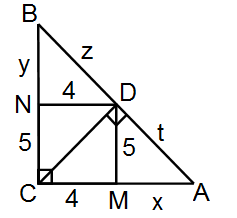CM || ND and CN || MD

Therefore CMDN is a rectangle

⇒ CM = ND = 4 and CN = MD = 5

In Δ CMD

By Pythagoras theorem

CD2 = CM2 + MD2

CD = √41

Also, Δ AMD ~ Δ DNB

⇒ y/5 = 4/x = z/t …….(i) {Sides of similar triangles are in proportion}

By Pythagoras Theorem

(x + 4)2 = 41 + t2 ……..(ii)

In Δ BDC

By Pythagoras Theorem

(y + 5)2 = 41 +z2 ………(iii)

From (ii) & (iii)

((x+4)2-41)/((y+5)2-41) = (t/z)2

((x+4)2-41)/((20/x)+5)2-41) = (x/4)2 {using (i)}

16 (x2 + 16 + 8x – 41) = 400 +25x2 + 200x – 41x2

4x2 – 9x – 100 = 0

x = 25/4 , y = 16/5 {using (i) again }

Hence AC = x + 4 = 41/4 and BC = 5 + y = 41/5.

Question 13: Let A, G and H be the arithmetic mean, geometric mean and harmonic mean, respectively of two distinct positive real numbers. If α is the smallest of the two roots of the equation A(G – H)x2 + G (H – A) x + H(A –G) = 0, then

1. a. –2 < α < –1
2. b. 0 < α < 1
3. c. –1 < α < 0
4. d. 1 < α < 2

Solution:

Given, A(G–H) x2 + G(H–A) x + H(A–G) = 0

Put x = 1 in above equation

A(G–H) + G(H–A) + H(A–G) = 0

0 = 0

⇒ x = 1 is one root (let it be ‘α’ and other root be ‘β’)

Now using product of roots,

α. β = A(A-G)/A(G-H)

1. β = (H.A-H.G)/A(G-H)

β = (G2-H.G)/A(G-H) {A.H = G2}

β = G(G-H)/A(G-H)

β = G/A < 1{ as A > G}

Question 14: In the figure, ABCD is a unit square. A circle is drawn with centre O on the extended line CD and passing through A. If the diagonal AC is tangent to the circle, then the area of the shaded region is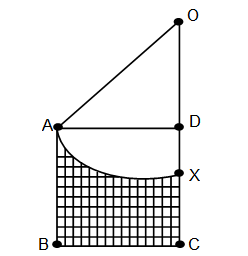1. a. (9-π )/6
2. b. (8-π )/6
3. c. (7-π )/4
4. d. (6-π )/4

Solution: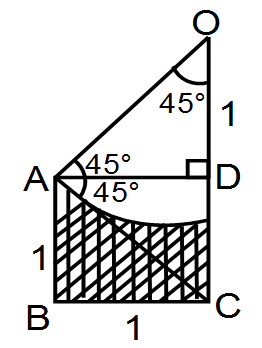AC is tangent to circle with radius OA

Therefore ∠ OAC = 900

Also, AC is diagonal of square

⇒ OA = AD cosec(450) = √2

Now, Required Area = Area of square – (area of sector OAX – area of Δ OAD)

= 1-( π(√2)2. ((π/4)/2 π)-(1/2)(1).(1))

= 1-((π/4)-(1/2))

= (3/2)-( π/4)

= (6-π )/4

Question 15: The sum of all non-integer roots of the equation x5 – 6x4 + 11x3 – 5x2 – 3x + 2 = 0 is

1. a. 6
2. b. –11
3. c. –5
4. d. 3

Solution:

x5 – 6x4 + 11x3 – 5x2 – 3x + 2 = 0

(x – 1) (x – 2) (x3 – 3x + 1) = 0 {factorizing}

Now, x3 – 3x + 1 has non integral roots whose sum is 3.

Question 16: Let S be the circle in xy-plane which touches the x-axis at point A, the y-axis at point B and the unit circle x2 + y2 = 1 at point C externally. If O denotes the origin, then the angle OCA equals

1. a. 5π/8
2. b. π/2
3. c. 3π/4
4. d. 3π/5

Solution: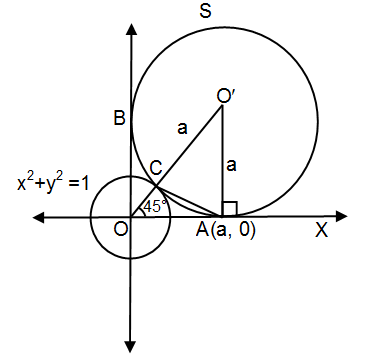Circle S touches both the axes, therefore its center lies on y = x, let it be O’(a,a).

So A(a,0) and B(0,a)

⇒ ∠ O’OA = 45

‘C’ lies on x2 + y2 = 1 & on OO’

Therefore C (1/√2, 1/√2)

OO’ = OC + CO’

√2a = 1+a

a = 1/√2-1 = √2+1

Now, slope of AC = (0-1/√2)/(1+√2-(1/√2)

= – 1/(√2+1)

= – (√2-1)

= – tan π/8

= tan (π –π /8)

= tan (7π /8)

So ∠ CAX = 7π/8

Also ∠ CAX = ∠ OCA + ∠ COA {Exterior angle property}

⇒ 7 π /8 = ∠ OCA + π /4

⇒ ∠ OCA = 5 π /8.

Question 17: In an isosceles trapezium, the length of one of the parallel sides, and the lengths of the non-parallel sides are all equal to 30. In order to maximize the area of the trapezium, the smallest angle should be

1. a. π/6
2. b. π/4
3. c. π/3
4. d. π/2

Solution: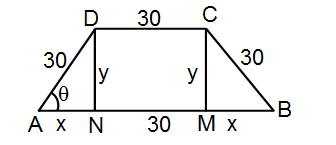x = 30 cos θ; y = 30 sin θ

Now, Area of trapezium

A = ½ (30+30+2x)×(y) { Area =1/2 (sum of parallel sides)height }

= (30+30cos θ) 30 sin θ

= 900 sin θ (1+cos θ)

Differentiating both sides with respect to θ

dA/d θ = 900 {sin θ. (– sin θ) +(1 + cos θ). cos θ } = 0 {for critical points}

⇒ cos2 θ – sin2 θ + cos θ = 0

⇒ 2cos2 θ + cos θ – 1 =0

⇒ (2cos θ – 1) (cos θ +1) = 0

⇒ cos θ = ½ or –1

θ = π /3 OR π (not possible).

Question 18: Let A1, A2, A3 be regions in the xy-plane defined by

A1 = {(x, y) : x2 + 2y2 ≤1},

A2 = {(x, y) : |x|3 + 2√2 |y|3 ≤ 1},

A3 = {(x, y) : max (|x|, √2 |y|) ≤1. Then

1. a. A1 ⊃A2 ⊃A3
2. b. A3 ⊃A1 ⊃A2
3. c. A2 ⊃A3 ⊃A1
4. d. A3 ⊃A2 ⊃A1

Solution:

$A_{1}=4\int_{0}^{1}\frac{{\sqrt{1-x^{2}}}}{\sqrt{2}}dx$ {ellipse}

= $\frac{4}{\sqrt{2}}\left \{ (\frac{x^{2}}{2}\sqrt{1-x^{2}}+\frac{1}{2}\sin ^{-1}x)_{0}^{1} \right \}$

= (4/√2)(0+π/4 -0)

= π/√2 ..(1)

Now for x(0,1)

x2> x3

1-x2<1-x3

$\sqrt{1-x^{3}}> \sqrt{1-x^{2}}$

Therefore A2 = $4\int_{0}^{1}\frac{\sqrt{1-x^{3}}}{\sqrt{2}}dx> 4\int_{0}^{1}\frac{\sqrt{1-x^{2}}}{\sqrt{2}}dx$ …(2)

= 2√2 ……..(3)

So from (1), (2) and (3)

A3 ⊃A2 ⊃A1

Question 19: Let ABCD be a square and E be a point outside ABCD such that E, A, C are collinear in that order. Suppose EB = ED = √130 and the areas of triangle EAB and square ABCD are equal. Then the area of square ABCD is

1. a. 8
2. b. 10
3. c. √120
4. d. √125

Solution: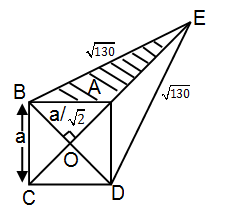Let side of square be ‘a’

⇒ Diagonal BD = a ⇒ OB = BD/2 = a/2

Now, In Δ OBE

BE2 = OE2+OB2 (using Pythagoras theorem)

⇒ OE = √130-a2/2

Now, ar (Δ EAB) = ar (Δ EOB) – ar (ΔAOB)

= (½)(a/√2) √(130-a2/2)-(1/2)(a/√2)2

= (a/4) √(130-a2/2)-a2/4

ar (square ABCD) = a2

Given, ar (Δ EAB) = ar (square ABCD)

⇒ (a2/4)√(260-a2)-a2/4 = a2

a2 (260 – a2) = 25a4

260 – a2 = 25a2

a2 = 10 (area of square).

Question 20: Consider the set A = {1, 2, 3, ..., 30}. The number of ways in which one can choose three distinct numbers from A so that the product of the chosen numbers is divisible by 9 is

1. a. 1590
2. b. 1505
3. c. 1110
4. d. 1025

Solution:

A = {1, 2, 3 …..….30}

Let X, Y , Z be subsets of A :

X = {9, 18, 27}

Y = {3, 6, 12, 15, 21, 24, 30}

Z = {1, 2, 4, 5, …….}

n(X) = 3, n(Y) = 7, n(Z) = 20

Now, the product of three selected numbers is divisible by 9, if

1) One from X, two from remaining

2) Two from X, one from remaining

3) All three from X

4) Two from Y, one from Z

5) All three from Y

So required no. of cases

= 3C1 ×27C2 +3C2 ×27C1 +3C3 + 7C2×20C1 +7C3

= 1590

### KVPY-SA 2017 Maths Paper with Solutions